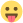• Viel Spass!

• Dann mal los

1. D2Lobo

Glück ist das einzige was sich verdoppelt, wenn man es teilt[SCHILD=random]der beste Lobo der Welt [/SCHILD]

• 2. F4Lobo

Glück ist das einzige was sich verdoppelt, wenn man es teilt[SCHILD=random]der beste Lobo der Welt [/SCHILD]

• 3. G1Lobo

Glück ist das einzige was sich verdoppelt, wenn man es teilt[SCHILD=random]der beste Lobo der Welt [/SCHILD]

• 4. G7Lobo

Glück ist das einzige was sich verdoppelt, wenn man es teilt[SCHILD=random]der beste Lobo der Welt [/SCHILD]

• 5. A1Lobo

Glück ist das einzige was sich verdoppelt, wenn man es teilt[SCHILD=random]der beste Lobo der Welt [/SCHILD]

• 6. A7Lobo

Glück ist das einzige was sich verdoppelt, wenn man es teilt[SCHILD=random]der beste Lobo der Welt [/SCHILD]

• 7. A4xD7Lobo

Glück ist das einzige was sich verdoppelt, wenn man es teilt[SCHILD=random]der beste Lobo der Welt [/SCHILD]

• 8. D5Lobo

Glück ist das einzige was sich verdoppelt, wenn man es teilt[SCHILD=random]der beste Lobo der Welt [/SCHILD]

• 9. C4Lobo

Glück ist das einzige was sich verdoppelt, wenn man es teilt[SCHILD=random]der beste Lobo der Welt [/SCHILD]

• 10. D2-D3Lobo

Glück ist das einzige was sich verdoppelt, wenn man es teilt[SCHILD=random]der beste Lobo der Welt [/SCHILD]